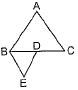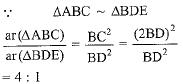Courses

# MCQ : Triangles - 1

## 10 Questions MCQ Test Mathematics (Maths) Class 10 | MCQ : Triangles - 1

Description
This mock test of MCQ : Triangles - 1 for Class 10 helps you for every Class 10 entrance exam. This contains 10 Multiple Choice Questions for Class 10 MCQ : Triangles - 1 (mcq) to study with solutions a complete question bank. The solved questions answers in this MCQ : Triangles - 1 quiz give you a good mix of easy questions and tough questions. Class 10 students definitely take this MCQ : Triangles - 1 exercise for a better result in the exam. You can find other MCQ : Triangles - 1 extra questions, long questions & short questions for Class 10 on EduRev as well by searching above.
QUESTION: 1

### In Fig. 7.4, ∠BAC = 90° and AD ⊥ BC. Then,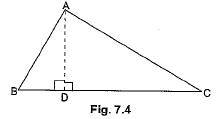Solution: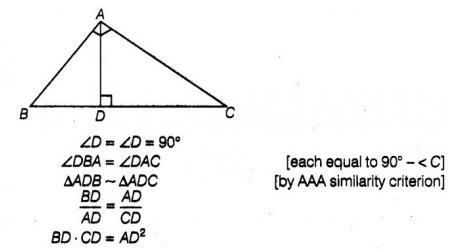QUESTION: 2

### In the given figure, AD/BD = AE/EC and ∠ADE = 70°, ∠BAC = 50°, then angle ∠BCA =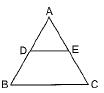Solution:

The correct option is Option D.

By using angle sum property, we find third angle

AED = 180° - (70°+50°) = 60°

and DE ll BC

So, by corresponding angle, we get the value of BCA = 60°.

QUESTION: 3

### If ΔABC ~ ΔEDF and ΔABC is not similar to ΔDEF, then which of the following is not true?

Solution:

Since △ABC~△EDF

Then, AB/ED = BC/DF = AC/EF

1) BC/DF = AC/EF

⇒BC.EF=AC.FD

So, A) is true

2) AB/ED = AC/EF

⇒AB.EF=AC.DE

So,B) is true

3) AB/ED = BC/DF

BC.DE=AB.EF

So, D) is true

Therefore, Option C is not true

QUESTION: 4

Which pair of the given quadrilaterals is similar?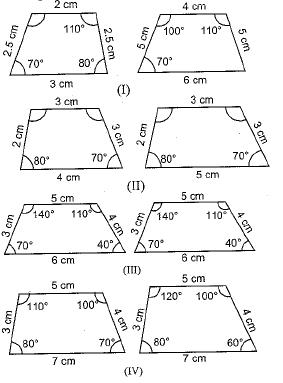Solution:

Quadrilaterals are similar if their angles are equal and corresponding sides are proportional.

QUESTION: 5

D and E are respectively the points on the sides AB and AC of a triangle ABC such that AD = 3 cm, BD = 5 cm, BC = 12.8 cm and DE || BC. Then length of DE (in cm) is

Solution:

GIVEN: In  Δ ABC, D and E are points on AB and AC , DE ||  BC and  AD = 2.4 cm, AE = 3.2 cm, DE = 2 cm and BE = 5 cm.
In Δ ADE and Δ ABC,
[DE || BC, AB is transversal]
∠AED =∠ACB     (corresponding angles)
[DE || BC, AC is transversal]
So, Δ ADE  ~ Δ ABC      (AA similarity)
Therefore, AD/AB = AE/AC = DE/BC
[In similar triangles corresponding sides are proportional]
2.4/(2.4+DB)  = 2/5
2.4 × 5  = 2(2.4+ DB)
12 = 4.8 + 2DB
12 - 4.8  = 2DB
7.2 = 2DB
DB = 7.2/2
DB = 3.6 cm
Similarly, AE/AC = DE/BC
3.2/(3.2+EC) = 2/5
3.2 × 5 = 2(3.2+EC)
16 = 6.4 + 2EC
16 - 6.4 = 2EC
9.6 = 2EC
EC = 9.6/2
EC = 4.8 cm
Hence,BD = 3.6 cm and CE = 4.8 cm.

QUESTION: 6

If in two triangles ABC and PQR, AB/QR = BC/PR = CA/PQ , then

Solution: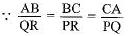Then, ΔCAB ~ ΔPQR

QUESTION: 7

If ΔPRQ ~ ΔXYZ, then

Solution:

Since the two triangles are similar, therefore, they will have their corresponding angles congruent and the corresponding sides in proportion.

QUESTION: 8

Match the column: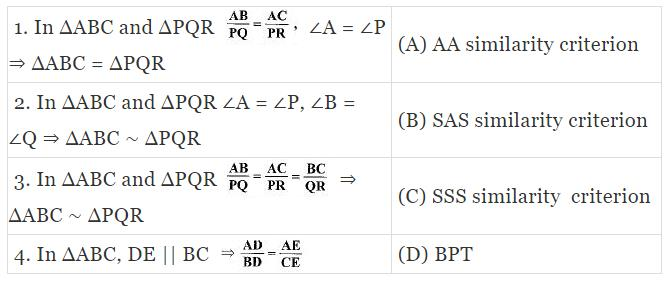Solution:

Properties of triangles.

QUESTION: 9

In triangles ABC and DEF, ∠B = ∠E, ∠F = ∠C and AB = 3DE. Then, the two triangles are

Solution:

to be congruent, the conditions are
S S S - three sides
S A S - two sides and the included angle
A S A - two angles and one side
R H S - R H S - Right angle, Hypotenuse and one side
But to be similar,
A A A means 3 angles
A A means only two angles ....
in both triangles should be equal.
In the problem, equality of two angles is given, but equality of sides is not given.
So, given triangles are not congruent.
But they are similar.

QUESTION: 10

ABC and BDE are two equilateral triangles such that D is mid-point of BC. Ratio of the areas of triangles ABC and BDE is

Solution: